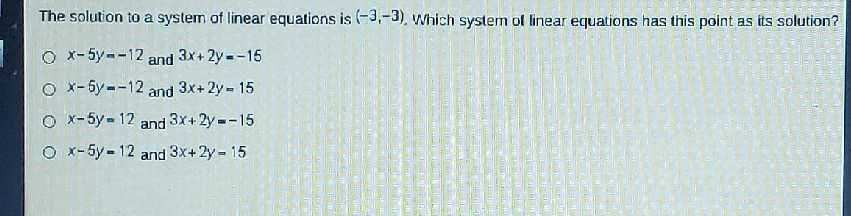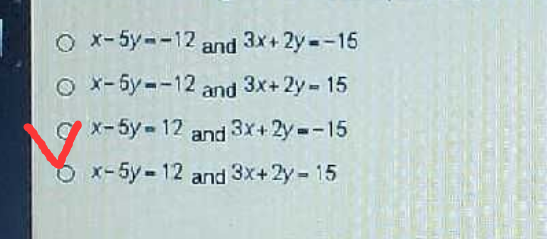### Still have math questions?

Algebra
QuestionThe solution to a system of linear equations is $$( - 3 , - 3 )$$ . Which system of linear equations has this point as its solution?

$$x - 5 y = - 12$$ and $$3 x + 2 y = - 15$$

$$x - 5 y = - 12$$ and $$3 x + 2 y = 15$$

$$x - 5 y = 12$$ and $$3 x + 2 y = - 15$$

$$x - 5 y = 12$$ and $$3 x + 2 y = 15$$